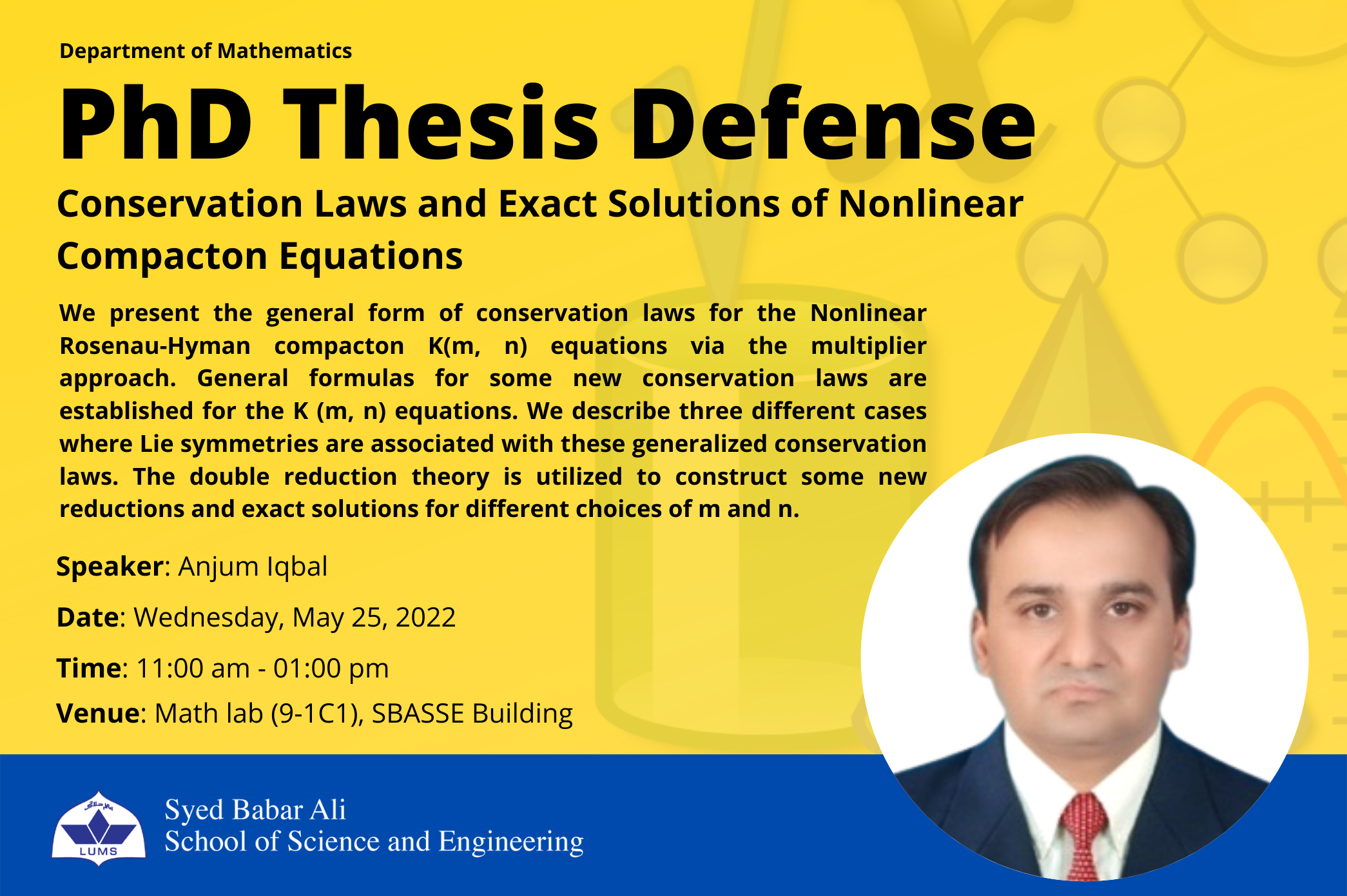Event date:
May 25 2022 11:00 am

## Conservation Laws and Exact Solutions of Nonlinear Compacton Equations

Supervisor
Dr. Imran Naeem
Student
Anjum Iqbal
Venue
Math lab (9-1C1)
Event
PhD Thesis defense

Abstract:

Compactons, or solitons with compact support, were first introduced as solutions of the nonlinear dispersive K (m, n) equation, which generalizes the celebrated KdV  equation. We present the general form of conservation laws for the nonlinear Rosenau-Hyman compacton K(m, n) equations via the multiplier approach. General formulas for some new conservation laws are established for the K (m, n) equations. We describe three different cases where Lie symmetries are associated with these generalized conservation laws. The double reduction theory is utilized to construct some new reductions and exact solutions for different choices of m and n. Next, we study a generalized equation that represents a family of compacton supporting equations. We present the general form of conservation laws for this family of  compacton equations, which results in the conservation laws, reductions, and exact solutions of certain subcases of the family equation. We also consider a generalized third-order nonlinear K (fm, gn) equation that generalizes the Rosenau-Hyman K(m,n) equation, extended Rosenau-Pikovsky K (cosm u, cosn u) equation, logarithmic KdV equation, generalized Gardner, and several other third-order nonlinear dispersive equations. Moreover, we obtain conservation laws of the K(fm, gn) equation in the most generalized form of m, n, f (u), and g (u). Several exact solutions of various equations of the K (fm, gn) form are derived from these generalized conservation laws by using the double reduction theory. The inclusion of the generalized K (fm, gn) equation as a central part of the KP equation results in a generalized KP-like equation KP (fm, gn), which for pertinent choices of m, n, f (u), and g (u) yields several variants of the KP equations, including the logarithmic KP-like equation, the generalized Gardner KP equation, and the KP equation with p-power non-linearity. We also derive the generalized conserved vectors for the KP (fm, gn)  equation in the form of unknown general functions f (u) and g (u) by employing the multiplier approach. For suitable m, n, f (u), and g (u) choices, the resulting conserved vectors are then utilized in the double reduction method to construct implicit and explicit exact solutions of various KP-like generalized equations. Finally, we present novel exact solutions for a slightly generalized form of Rosenau's quintic dispersive equation. The double reduction theory is utilized to obtain fourth-order nonlinear ODE, which is then solved to compute exact solutions for the quintic equation. In particular, the (G'/G)-expansion approach is employed to procure new exact solutions for the quintic PDE with cubic nonlinearity by using the reduced fourth-order nonlinear ODE. The structure of these solutions is analyzed by some graphs that illustrate soliton and compacton solutions for different parameter values.

Final Defense Committee (FDC):

Dr. Amer Rasheed (Convener of committee)

Dr. Naveed ul Hassan (External Member, EE-SSE)

Dr. Sultan Sial (Member)

Dr. Imran Naeem (Supervisor)

Dr. Fiazud Din Zaman (Examiner, ASSMS)

Publications:

1). A. Iqbal, I. Naeem, Generalised conservation laws, reductions and exact solutions of the K (m, n) equations via double reduction theory, Pramana 95 (1) (2021) 1 - 9 (Springer, Impact Factor: 2.219).

2). A. Iqbal, I. Naeem, Generalized compacton equation, conservation laws and exact solutions, Chaos, Solitons & Fractals (2021) 111604 (Elsevier, Impact Factor: 5.944).

3). A. Iqbal, I. Naeem, Conservation Laws and Exact Solutions of a Family of Compacton Supporting Equations, The European Physical Journal Plus, vol. 137, no. 5, pp. 1-9, 2022 (Springer, Impact Factor: 3.911).

4). A. Iqbal, I. Naeem, Conservation Laws and Exact Solutions of a Generalized KP-like Equations, (Accepted article) Mathematical Methods in the Applied Sciences,

(John Wiley and Sons , Impact Factor: 2.321).

5). A. Iqbal, I. Naeem, Exact Solutions of a Quintic Dispersive Equation, (Revision Submitted to Chaos, Solitons & Fractals (Elsevier, Impact Factor: 5.944).